# 模型评估与改进（三）// 评估指标

1、常用的评估指标有哪些

• 第一部分：二分类的评估指标

2、精度accuracy指标对评估二分类模型的不足？

3、混淆矩阵confusion matrix是什么？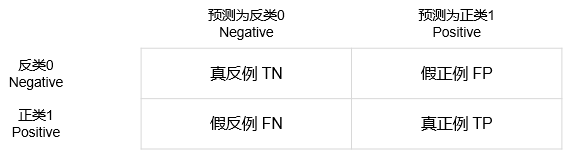4、由混淆矩阵引申的一些常用指标？

f-分数f-score=2*（precision*recall）/（precision+recall），是准确率和召回率的调和平均；

5、如何评估预测的不确定性？

6、准确率-召回率曲线precision_recall_curve是什么？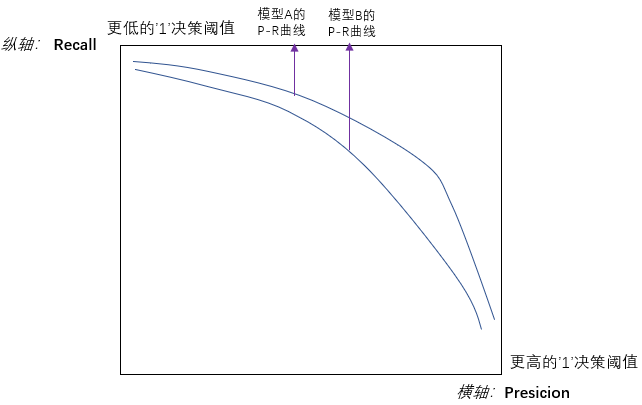7、ROC曲线roc_curve是什么？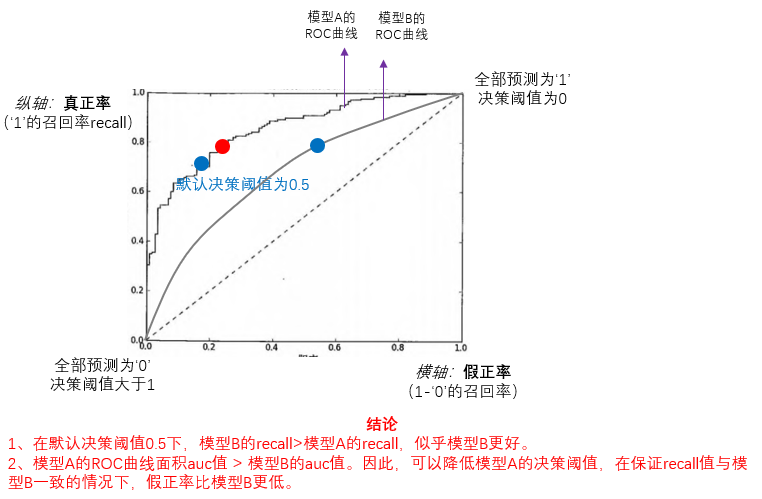8、二分类评估指标的常用sklearn库？

``````from sklearn.metrics import confusion_matrix
from sklearn.metrics import f1_scorefrom sklearn.metrics import classification_report #查看precision 、recall 、f1-score 、support值
from sklearn.metrics import classificationfrom sklearn.metrics import precision_recall_curve #准确率-召回率曲线
from sklearn.metrics import average_precision_score #平均准确率，即准确率-召回率曲线下面积from sklearn.metrics import precision_recall_fscore_support
from sklearn.metrics import precision_scorefrom sklearn.metrics import roc_curve #ROC曲线（假正率-真正率曲线）
from sklearn.metrics import roc_auc_score #AUC，即ROC曲线下面积
``````
• 第二部分：多分类的评估指标

9、精度accuracy指标对评估多分类模型的不足？

10、如何有效评估多分类模型？

11、多分类评估指标的常用sklearn库？

``````from sklearn.metrics import confusion_matrix
from sklearn.metrics import classification_report #查看precision 、recall 、f1-score 、support值
``````
• 第三部分：回归的评估指标

12、回归的评估指标有哪些？

R-squared = SSR/SST=1-SSE/SST
（SST=SSR+SSE，SST(total sum of squares)为总平方和，SSR(regression sum of squares)为回归平方和，SSE(error sum of squares) 为残差平方和）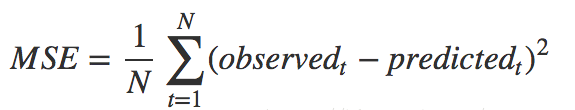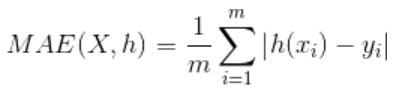• 第四部分：设置评估指标

13、如何在python实现设置评估指标？